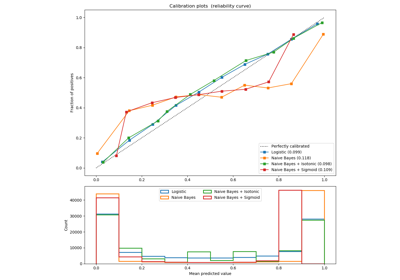# sklearn.metrics.f1_score¶

sklearn.metrics.f1_score(y_true, y_pred, labels=None, pos_label=1, average=’binary’, sample_weight=None)[source]

Compute the F1 score, also known as balanced F-score or F-measure

The F1 score can be interpreted as a weighted average of the precision and recall, where an F1 score reaches its best value at 1 and worst score at 0. The relative contribution of precision and recall to the F1 score are equal. The formula for the F1 score is:

F1 = 2 * (precision * recall) / (precision + recall)


In the multi-class and multi-label case, this is the average of the F1 score of each class with weighting depending on the average parameter.

Read more in the User Guide.

Parameters: y_true : 1d array-like, or label indicator array / sparse matrix Ground truth (correct) target values. y_pred : 1d array-like, or label indicator array / sparse matrix Estimated targets as returned by a classifier. labels : list, optional The set of labels to include when average != 'binary', and their order if average is None. Labels present in the data can be excluded, for example to calculate a multiclass average ignoring a majority negative class, while labels not present in the data will result in 0 components in a macro average. For multilabel targets, labels are column indices. By default, all labels in y_true and y_pred are used in sorted order. Changed in version 0.17: parameter labels improved for multiclass problem. pos_label : str or int, 1 by default The class to report if average='binary' and the data is binary. If the data are multiclass or multilabel, this will be ignored; setting labels=[pos_label] and average != 'binary' will report scores for that label only. average : string, [None, ‘binary’ (default), ‘micro’, ‘macro’, ‘samples’, ‘weighted’] This parameter is required for multiclass/multilabel targets. If None, the scores for each class are returned. Otherwise, this determines the type of averaging performed on the data: 'binary': Only report results for the class specified by pos_label. This is applicable only if targets (y_{true,pred}) are binary. 'micro': Calculate metrics globally by counting the total true positives, false negatives and false positives. 'macro': Calculate metrics for each label, and find their unweighted mean. This does not take label imbalance into account. 'weighted': Calculate metrics for each label, and find their average weighted by support (the number of true instances for each label). This alters ‘macro’ to account for label imbalance; it can result in an F-score that is not between precision and recall. 'samples': Calculate metrics for each instance, and find their average (only meaningful for multilabel classification where this differs from accuracy_score). sample_weight : array-like of shape = [n_samples], optional Sample weights. f1_score : float or array of float, shape = [n_unique_labels] F1 score of the positive class in binary classification or weighted average of the F1 scores of each class for the multiclass task.

Notes

When true positive + false positive == 0 or true positive + false negative == 0, f-score returns 0 and raises UndefinedMetricWarning.

References

Examples

>>> from sklearn.metrics import f1_score
>>> y_true = [0, 1, 2, 0, 1, 2]
>>> y_pred = [0, 2, 1, 0, 0, 1]
>>> f1_score(y_true, y_pred, average='macro')
0.26...
>>> f1_score(y_true, y_pred, average='micro')
0.33...
>>> f1_score(y_true, y_pred, average='weighted')
0.26...
>>> f1_score(y_true, y_pred, average=None)
array([0.8, 0. , 0. ])


## Examples using sklearn.metrics.f1_score¶Probability Calibration curves